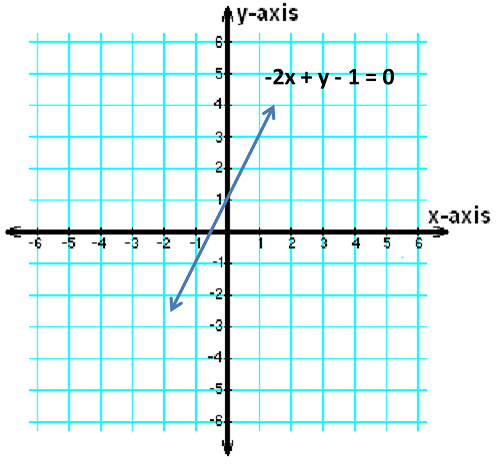# Standard Form: Equations & Formulas

Instructor: Kimberlee Davison

Kim has a Ph.D. in Education and has taught math courses at four colleges, in addition to teaching math to K-12 students in a variety of settings.

Standard form is one of many ways to write the equation of a line. In this lesson, you'll learn how to write a line in standard form, and we'll discuss why it's important.

## Equations

The standard form is simply a particular format for writing an equation of a line. It looks like this:

Ax + By + C = 0

The key is that the x part comes first, the y part comes next, and the constant, or C (the number without an 'x' or 'y'), comes last.

For example, if A = 2, B = 3 and C = 3, the standard form would look like:

2x + 3y + 3 = 0

## Why Standard Form Matters

Looking at equations in standard form can be very helpful. In mathematics, when an equation is a line, it simply means that you have two things that vary - maybe the number of swings on a playground at the local elementary school and the number of Band-Aids the principal needs for students who fall off the swings in the month of October. But, more importantly, those two things have a consistent and precise relationship. If there are 2 swings, the principal will need 13 Band-Aids in October. If there are 5 swings, he will need 25. If there are 9 swings, he will need 41.

Given these numbers, the relationship between Band-Aids and swings may seem a little confusing. In fact, you may wonder whether there is a consistent relationship at all. A verbal description of the rule used to get from swings to Band-Aids may help: multiply the number of swings by 4 and add 5 to get the number of Band-Aids needed. Stating the rule clarifies the relationship.

Another way you might clarify the relationship is like this:

Band-Aids = 4 x swings + 5

or

Band-Aids - 5 = 4 x swings

or

Band-Aids - 4 x swings = 5

or

- 4 swings + Band-Aids - 5 = 0

or

-4 x+ y - 5 = 0 (where x represents swings and y represents Band-Aids).

All of those four equations represent the same relationship. Nothing is wrong with any of them. The last one is in standard form.

Why does this matter? Well, pretend there are two schools in your neighborhood - Oak School and Maple School. Oak School's swing to Band-Aid formula looks like this:

y = 2x + 1

Maple School's formula looks like this:

-2x+ y = 1

Are they the same, or are they different? With a little algebra, you would discover that they are the same - just rearranged. When graphed, they both indicate the line shown.To unlock this lesson you must be a Study.com Member.
Create your account

### Register to view this lesson

Are you a student or a teacher?

### Unlock Your Education

#### See for yourself why 30 million people use Study.com

##### Become a Study.com member and start learning now.
Back
What teachers are saying about Study.com
Create an account to start this course today
Used by over 30 million students worldwide